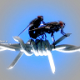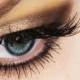### bullets

1.buldy

doamne cat am ras, e de toata jena pisica asta. super filmuletz

2.RazvanCristian

=))))))))))))))
zici ca a vrut sa isi ia zborul mai mult decat sa sara =)))))
SUPERCAAAAAAAATTTTTTTTT =)))

3.roxi

=)) =))=))=))

4.Sizzling ca la aparate